Module 4 - Supply Flashcards Preview

Economics > Module 4 - Supply > Flashcards

Flashcards in Module 4 - Supply Deck (43):
1

2

3

4

5

6

7

8

9

Figure 4.26 provides information aboutI. engineering-inefficient outputs. II. economic efficiency, since wheat is always in demand because of world food shortages.Which of the following is correct?A. I only. B. II only. C. Both I and II. D. Neither I nor II.10

From Figure 4.26 it can be concluded thatI. points X, Y and Z are the only attainable outputs.II. B is an attainable output.III. A is an unattainable output even in the long run. Which of the following is correct?A. I only. B. II only. C. III only. D. Not I, nor II nor III.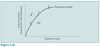11

12

13

14

15

16

17

18

A recent economics graduate was hired by a highly competitive restaurant and was assigned the task of improving efficiency in the restaurant. It was felt that an inefficient combination of two variable factor inputs was being employed. The economist was able to make the following calculations where each lunch sells for \$2.00:The economist correctly diagnosed the problem and advised the restaurant owner on the appropriate course of action. That course was to:A. hire more A and more B. B. hire more A but no more B. C. hire more B but no more A. D. hire less B and less A.19

The higher theI. marginal product of a factor input, the lower the marginal cost of output.II. average product of a factor input, the lower the average cost of output.III. costs of fixed factor inputs, the lower the marginal cost of output.Which of the following is correct?A. I only. B. III only. C. I and II only. D. I, II and III.

The correct answer is C. Suppose the factor input in question is labour, the variable factor input; then, with Q = output and L = units of labour, we have{image}where W = wage rates and the other expressions refer to total, fixed and variable costs.The expression Q/L appears in Equation A2.1 and L/Q appears in Equation A2.3. Thus APL and AC are inversely related. Thus II in the question is correct. ΔQ/ΔL appears in Equation A2.2 and ΔL/ΔQ appears in Equation A2.4. Thus MPL and MC must be inversely related, and so I in the question is correct. ΔFC/ΔQ = 0, and thus fixed cost and MC must be unrelated. Therefore III in the question is incorrect.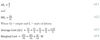20

21

22

23

24

Any point on the production frontier identifies outputI. produced in a technically efficient manner II. produced in an economically efficient mannerWhich of the following is correct? A. I only B. II only C. Both I and II D. Neither I nor II25

Which unit of labour has the highest marginal product (MPL)?A. 3 B. 5 C. 4 D. 626

Consider points I. 25,8 II. 25,9 III. 25,10 IV. 25,11Which of the following output/factor combinations is attainable? A. I only B. II only C. II, III and IV only D. I, II, III and IV27

Consider points I. 25,8 II. 25,9 III. 25,10 IV. 25,11Which of these points is attainable and engineering efficient? A. I only B. II only C. II, III and IV only D. I, II, III and IV28

Consider points I. 25,8 II. 25,9 III. 25,10 IV. 25,11The fixed factor inputs are altered slightly and the production frontier shifts upwards; point 25,8 is on the new frontier.Which of the following is correct? The engineering efficient outputs now areA. I only B. IV only C. II, III and IV only D. III only29

What does the area under the curve equal? A. The marginal product of labour B. The average product of labour C. Total product D. The optimal amount of labour to employ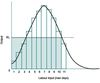30

If the going wage rate fell below W1, what would happen to the total wage bill (W) and the total returns to all other factors inputs (R)?A. W would increase, R would decrease B. W would increase, R would increase C. W might increase or decrease, R would increase D. W might increase or decrease, R would decrease31

32

33

34

At what level of output (Q) is average productivity of labour (APL) greatest?A. 19 B. 22 C. 24 D. 25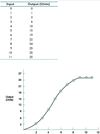35

At points 15,5 and 24,8 theI. average products of labour (APL) are equal. II. marginal products of labour (MPL) are equal.Which of the following is correct? A. I only. B. II only. C. Both I and II. D. Neither I nor II.36

The marginal product (MPL) of the third unit of labour is 3, i.e.△Q/△L = 6-3/1At what other point of the production frontier does MPL= 3? A. 10,4 B. 15,5 C. 22,7 D. 19,637

Which of the following is correct?I. When MPL is decreasing, APL is decreasing also II. When MPL is at a maximum, APL is at a maximum alsoA. I only B. II only C. Both I and II D. Neither I nor II38

Which of the following is correct?I. If APL > 0,TP > 0 II. If APL > 0, MPL > 0A. I only B. II only C. Both I and II D. Neither I nor II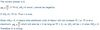39

An inverse relationship exists between certain productivity and cost variablesI. when MPL is minimum, AVC is minimum II. when APL is maximum, AVC is minimumWhich of the following is correct? A. I only B. II only C. Both I and II D. Neither I nor II40

As additional units of labour were hiredI. MPL was decreasing but APL was increasing II. MC was rising but AVC was decreasingWhich of the following is correct?A. Only I could occur B. Only II could occur C. Both I and II could occur D. Neither I nor II could occur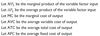A decreasing MPL and an increasing APL are possible simultaneously; as long as MPL>APL, APL will increase. When MPLL, APL will decrease. Thus I is true. Since MPL is inversely related to MC and since APL is inversely related to AVC a mirror image of the MPL/APL relationship will be reflected in the MC/AVC relationship. Thus II is true.

4142

43